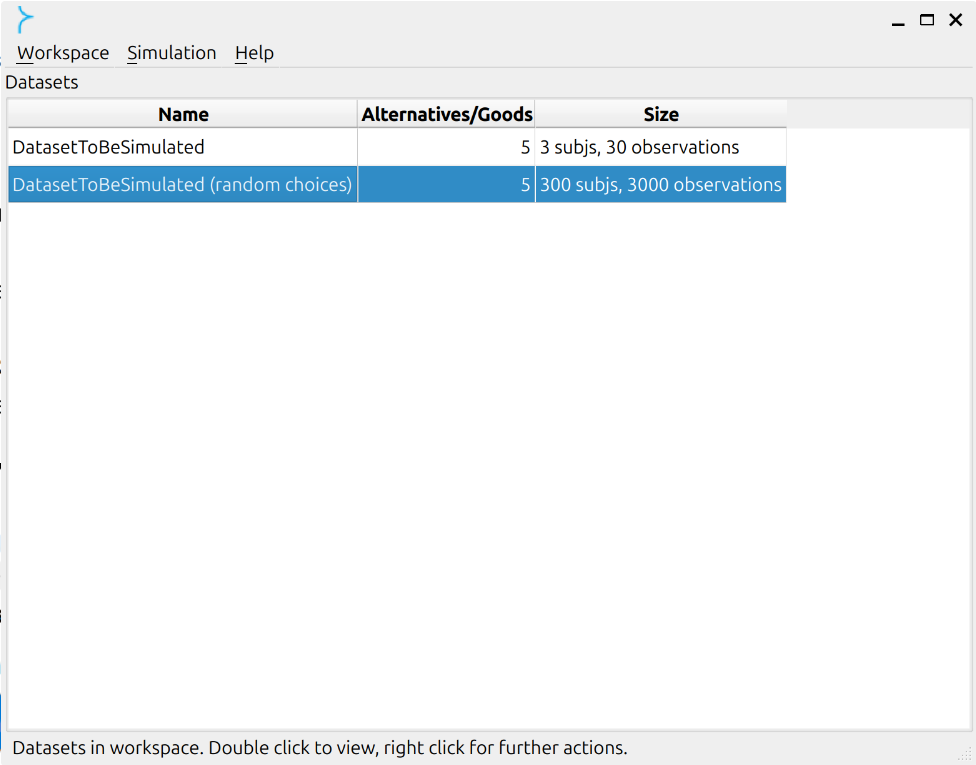# Simulations¶

Prest offers two ways in which you can obtain information about the probability distribution of various variables of interest (e.g. axiom violations; model distance scores) when a large number of artificial subjects are assumed to make uniform-random choices from menus that are derived from a finite set of general choice alternatives.

From these probability distributions you can then identify human subjects whose choice behaviour cannot be distinguished from random behaviour for a given level of statistical significance.

This procedure therefore allows you to perform a power test for your model-estimation and consistency-analysis computations on general datasets, as was first suggested in Bronars  for budgetary datasets.

## Generating random datasets and choices¶

Click on “Simulation -> Generate random subjects…”: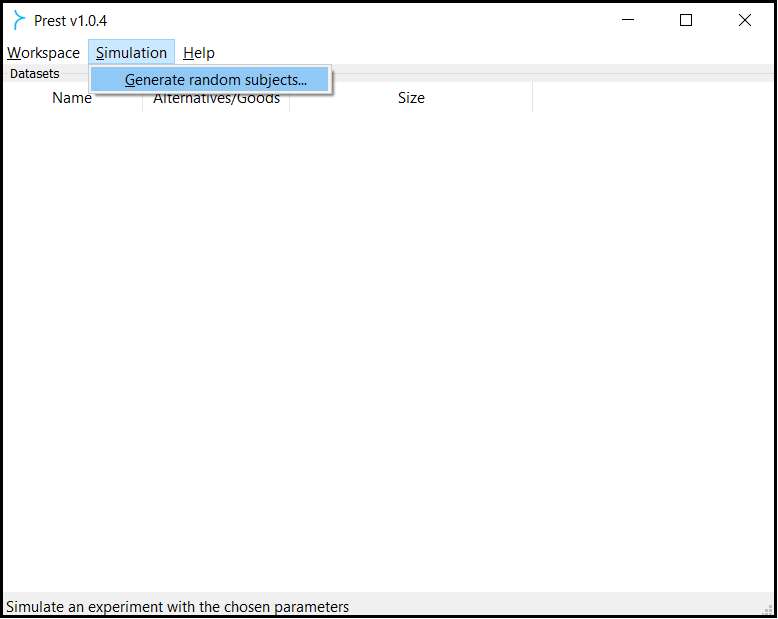Name the simulated dataset to be created, specify the number and labels of the choice alternatives
(separated by commas), and choose the desired number of artificial, random-behaving subjects: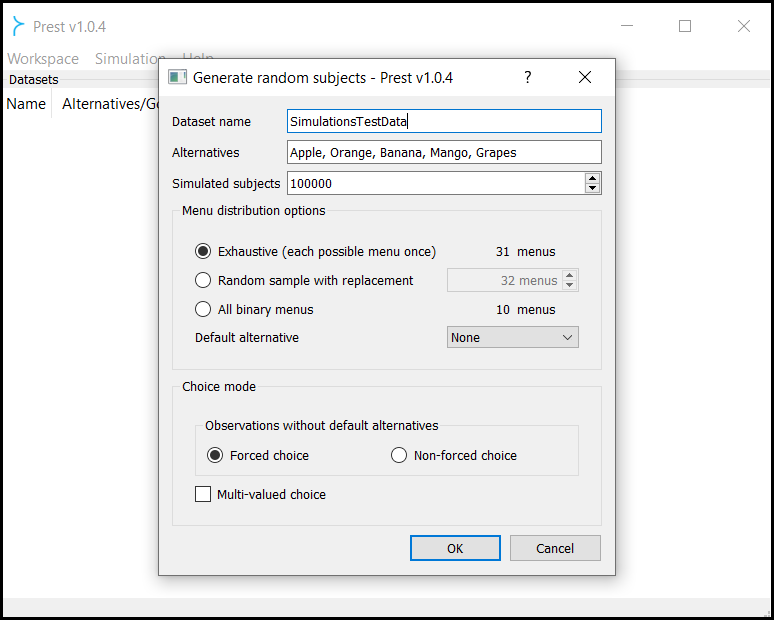Under “Menu distribution options” select one of the following:

• “Exhaustive (each possible menu once)”. Choices are made from all $$2^n-1$$ menus that are derived from the specified set with $$n$$ elements.

• “Random sample with replacement”. Choices are made from a random selection of the $$2^n-1$$ menus that are derived from the specified set with $$n$$ elements, possibly with repetitions.

• “All binary menus”. Choices are made from the $${n}\choose{2}$$ binary menus that are derived from the underlying set with $$n$$ alternatives.

• “Default alternative”. Select between “None” and “Uniformly random”; in the latter case every feasible alternative in every menu is equally likely to be the default.If you selected “Default alternative -> None” above, then you can also select one of the following under “Choice mode”/”Observations without default alternatives”:

• “Forced choice”: some alternative is chosen from every menu (deferral/outside option not feasible).

• “Non-forced choice”: choices can be empty-valued (deferral/outside option feasible).

Note

In both these cases you can allow multiple alternatives to be chosen by checking the “Multi-valued choice” box.

The corresponding simulated choice probabilities are as follows:

Menu with k alternatives

Single-valued choice

Multi-valued choice

$$\qquad\qquad\qquad\qquad\qquad\qquad\qquad\qquad\qquad$$ Forced choice

Probability for any alternative

$$\frac{1}{k}$$

$$\frac{1}{2}\frac{2^k}{2^k-1}$$

Not defined

$$\frac{1}{2^k-1}$$

$$\qquad\qquad\qquad\qquad\qquad\qquad\qquad\qquad\qquad$$ Non-forced choice

Probability for any alternative (excluding deferral/outside option)

$$\frac{1}{k+1}$$

$$\frac{1}{2}\frac{2^k}{2^k-1}\frac{k}{k+1}$$

Probability for deferral/outside option

$$\frac{1}{k+1}$$

$$\frac{1}{k+1}$$

Not defined

$$\frac{1}{2^k-1}\frac{k}{k+1}$$

If you selected “Default alternative -> Uniform” above, then you can also select one of the following under “Choice mode”/”Observations with default alternatives”:

• “Unbiased”: all alternatives (including the default) are equally likely to be chosen.

• “Default-biased”: this adapts the structure of “Non-forced choice” simulations to an environment where a default/status quo option is present and replaces the deferral/outside option; however, because the default/status quo option is one of the $$k$$ alternatives in the menu now, this adaptation generates a choice probability distribution that is biased towards that option.

Note

In both these cases you can again allow multiple alternatives to be chosen by checking the “Multi-valued choice” box.

In this case the corresponding simulated choice probabilities are as follows:

Menu with k alternatives

Single-valued choice

Multi-valued choice

$$\qquad\qquad\qquad\qquad\qquad\qquad\qquad\qquad\qquad$$ Unbiased

Probability for any alternative except the default/status quo option

$$\frac{1}{k}$$

$$\frac{1}{2}\frac{2^k}{2^k-1}$$

Probability for the default/status quo option

$$\frac{1}{k}$$

$$\frac{1}{2}\frac{2^k}{2^k-1}$$

Not defined

$$\frac{1}{2^k-1}$$

$$\qquad\qquad\qquad\qquad\qquad\qquad\qquad\qquad\qquad$$ Default-biased

Probability for any alternative except the default/status quo option

$$\frac{1}{k+1}$$

$$\frac{1}{2}\frac{2^k}{2^k-1}\frac{k}{k+1}$$

Probability for the default/status quo option

$$\frac{2}{k+1}$$

$$\frac{1}{k+1}+\frac{1}{2}\frac{2^k}{2^k-1}\frac{k}{k+1}=\frac{2^kk+2(2^k-1)}{2(2^k-1)(k+1)}$$

Not defined

$$\frac{k}{(k+1)(2^k-1)}$$

Note

“Single-valued choice” here refers to the case where “Multi-valued choice” at the bottom of the dialog box is not selected, and results in up to one alternative being chosen from each menu.

Note

The probability of an alternative being chosen under the “Multi-valued choice” mode is interpreted as the probability that this belongs to the chosen submenu of the relevant menu. Assuming “Forced choice” and considering an arbitrary menu $$A$$ with $$k$$ alternatives, every nonempty weak submenu of $$A$$ is chosen with probability $$\frac{1}{2^k-1}$$. Since each of the $$k$$ alternatives belongs to exactly $$\frac{2^k}{2}$$ of these submenus, it follows that each of them is chosen with probability $$\frac{2^k}{2(2^k-1)}$$. If “Non-forced choice” is selected instead, then since some nonempty submenu of $$A$$ is chosen with probability $$\frac{k}{k+1}$$ (because the deferral/outside option is chosen with probability $$\frac{1}{k+1}$$), the corresponding choice probability for each of the $$k$$ alternatives is adjusted accordingly.

In all cases the resulting random dataset will appear in the workspace and you can apply any Prest operation on it.
The simulated subjects are named “Random1, Random2, …”.
You can inspect individual entries by double-clicking on the dataset.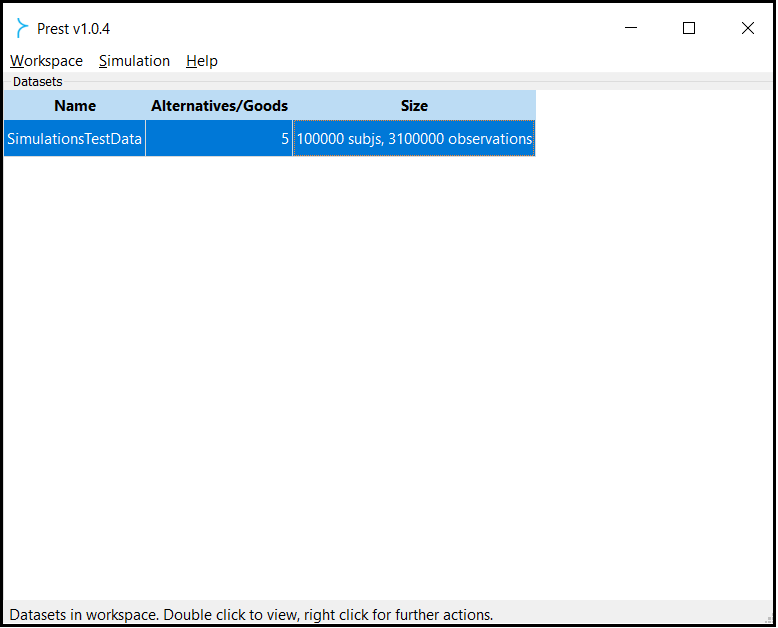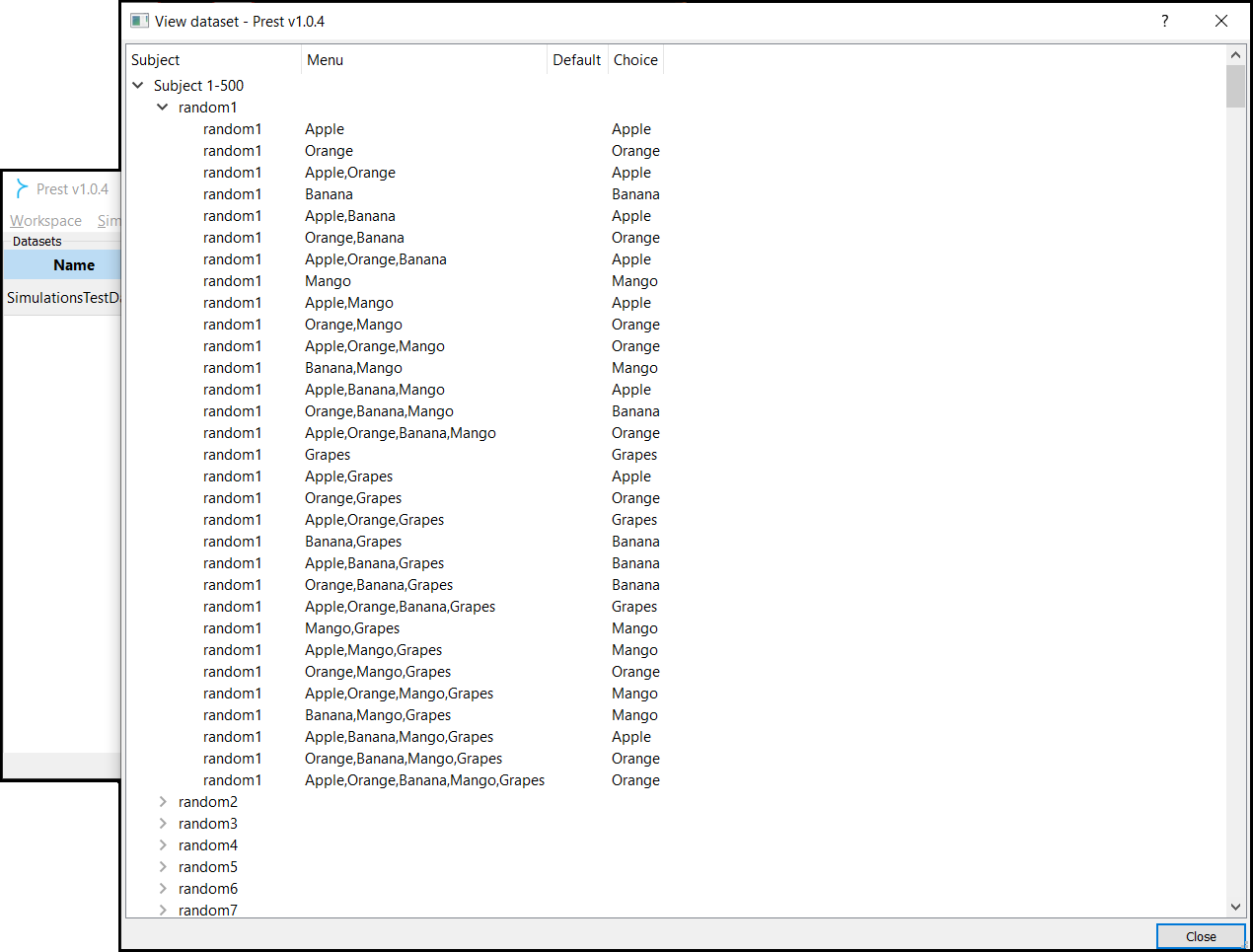## Generating random choices based on an existing dataset¶

You can use this feature to generate choices of random-behaving subjects who faced exactly the same menus that subjects
in an existing dataset were presented with.

In this case, Prest reproduces subject-by-subject the menu structure of the original dataset.

To do so, right-click on the relevant dataset in the workspace area and select “Analysis -> Generate similar random dataset”.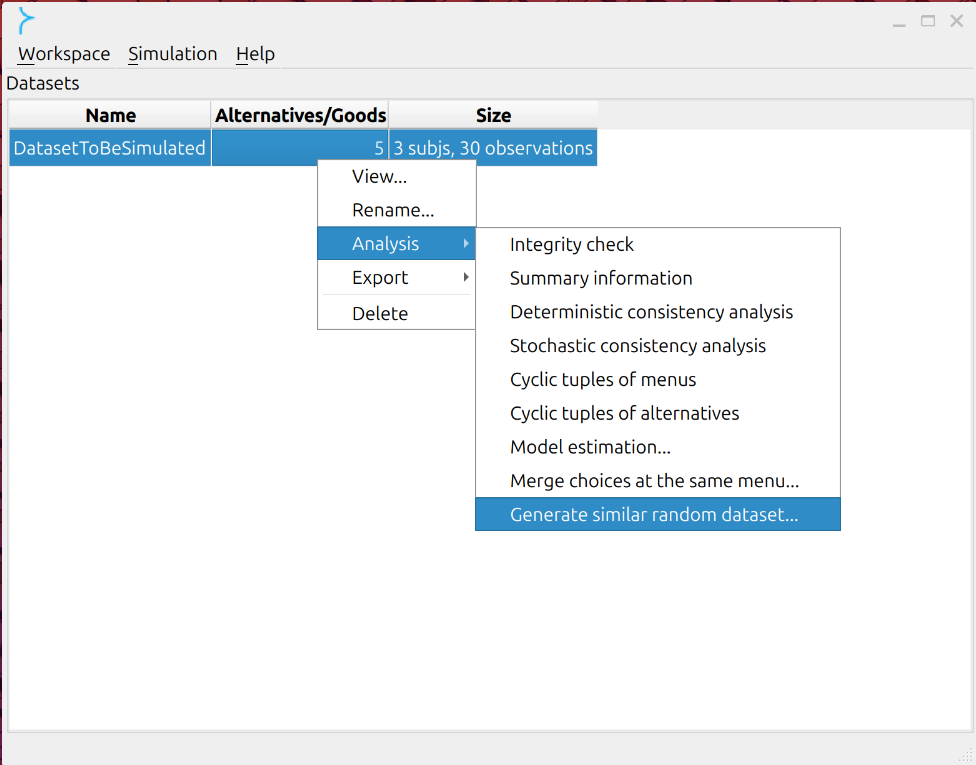In the pop-up window, the “Random subjects per subject” option specifies how many simulated subjects will be generated
in the way described above for each subject in the original dataset.

The “Subjects” and “Observations” entries below that option enable you to fine-tune the size dimensions
of the simulated dataset that will be produced.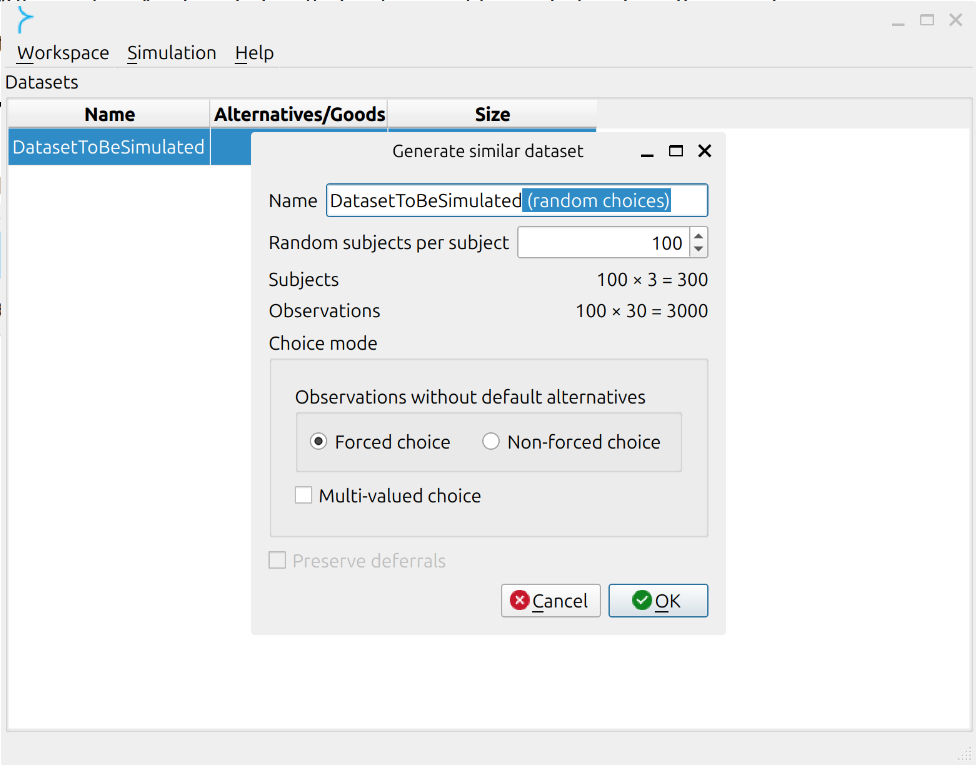The options that were specified above are also available here under “Choice mode”.

If the existing dataset contains some observations with default alternatives and others without
(see, for example, the hybrid dataset), then you can configure the simulation for each mode of analysis.

If the existing dataset contains some observations where the deferral/outside option was chosen,
then you can check the “Preserve deferrals” box to ensure that the simulated datasets
also feature choice of the deferral/outside option at all relevant menus.

The resulting random dataset will again appear in the workspace and you can apply any Prest operation on it.

The simulated subjects here are named
“Subject1Random1, …, Subject1RandomN, SubjectKRandom1, …, SubjectKRandomN”,
where “Subject1, …, SubjectK” are the subjects’ names in the original dataset
and $$N$$ is the number of simulated subjects selected by you.